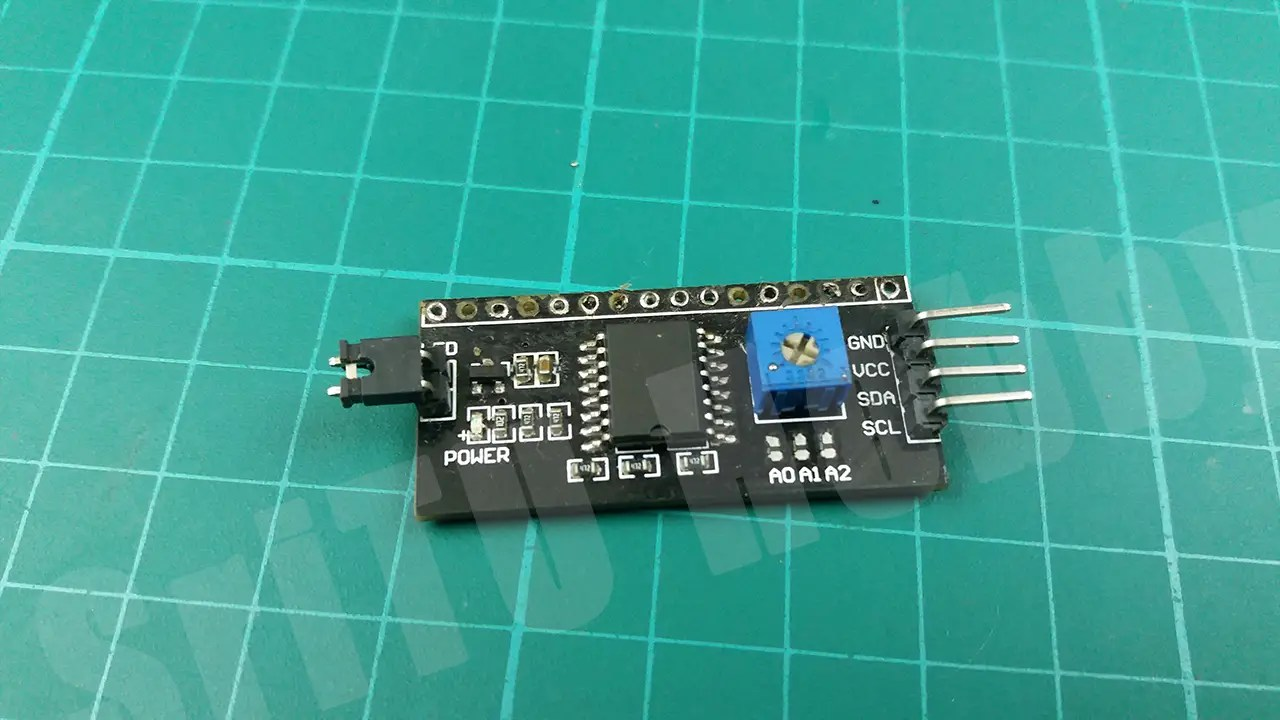# WATER LEVEL SENSOR Tutorial| How to use WATER LEVEL SENSOR

##Hello guys, welcome back to another tutorial from SriTu Hobby. This tutorial includes how does work the Water level sensor with Arduino. Also, this tutorial covers how to measure water levels using a water level sensor and display those values on the LCD Display. We can monitor factors such as water levels, leaks, and rainfall through this sensor.### How the water level sensor works.

In this sensor, we can see 10 copper straps. 5 of them are power straps and the other 5 are sensory straps. This sensor also works in the same way as the Rain sensor and Soil moisture sensor we have presented in previous articles. You can study them at the links below.

Soil moisture Sensor — Read it

This water level sensor also acts as a variable resistor. The resistance varies according to the amount of water. The resistance value is inversely proportional to the height at which the sensor is submerged in water. When the sensor is submerged in more water, it has better conductivity and less resistance. Also, when the sensor is submerged in low water, it has a higher resistance due to its weak conductivity.
In this sensor, we can see three main pins. They are described below.
• Signal – Through this we can get the water level as an analog value.
• VCC – This pin should have a potential of 3.3v to 5v.
• GND – Ground of this sensor.Well, now we will learn how this sensor works with Arduino. Below are the accessories required for this.
OK, Let’s do this project step by step.

### Step 1

Identify these components.
Arduino UNO board.Water level sensor.LCD Display.I2C Module.Jumper wires.### Step 2

Connect these components using the circuit diagram below.### Step 3

Now let us create the required program for this. First, download the I2C library required to control the LCD display and include it into the Arduino IDE.
The required code for this is given below.
``````#include <LiquidCrystal_I2C.h>
LiquidCrystal_I2C lcd(0x27, 16, 2);
void setup() {
Serial.begin(9600);
lcd.init();
lcd.backlight();
}
void loop() {
lcd.setCursor(0, 0);
lcd.print("Value :");
lcd.print(value);
lcd.print("   ");
Serial.println(value);
lcd.setCursor(0, 1);
lcd.print("W Level :");

if (value == 0) {
lcd.print("Empty ");
} else if (value > 1 && value < 350) {
lcd.print("LOW   ");
} else if (value > 350 && value < 510) {
lcd.print("Medium");
} else if (value > 510){
lcd.print("HIGH  ");
}
}``````

#### code explanation

This code contains the I2c library first. Then create an object as “lcd” for that library and give the I2c address and the length and width of the LCD display we are using.
#include <LiquidCrystal_I2C.h>
LiquidCrystal_I2C lcd(0x27162);

Then serial monitor and I2C communication are started in the void setup. Also, the backlight of the LCD display is turned on.
void setup() {
Serial.begin(9600);
lcd.init();
lcd.backlight();
}
In the void loop, “anlogRead” reads the sensor value and inserts it into the variable “value”. It then displays on the LCD display and the serial monitor.
lcd.setCursor(00);
lcd.print(“Value :”);
lcd.print(value);
lcd.print(”   “);
Serial.println(value);
lcd.setCursor(01);
lcd.print(“W Level :”);
Finally, the value is checked in IF condition and the LCD display is 0 if it is “empty”, 0-350 is “low”, 350-510 is “medium” and 510 is “high”.
if (value == 0) {
lcd.print(“Empty “);
} else if (value > 1 && value < 350) {
lcd.print(“LOW   “);
} else if (value > 350 && value < 510) {
lcd.print(“Medium”);
} else if (value > 510){
lcd.print(“HIGH  “);
}
Now upload this code to the Arduino board. First, select the correct board and port and then upload.After uploading the code correctly, we can test this project. Use a pot of water for that. You can watch it in the video below. We will meet in the next tutorial.

WATER LEVEL SENSOR Tutorial| How to use WATER LEVEL SENSOR

### 3 thoughts on “WATER LEVEL SENSOR Tutorial| How to use WATER LEVEL SENSOR”

1. what if I got this error? avrdude: ser_open (): can’t set com-state for “\\. \ COM5”

1. Please select the correct COM port.

2. I want to add a 5v water pump to this setup that starts when the water level sensor is 50 and stops when the water level is 450. Can you help with that?

Shopping Cart
Scroll to Top